### Home > CC4 > Chapter 10 > Lesson 10.2.1 > Problem10-79

10-79.
1. Given each pair of functions below, describe how the second function is transformed from the first function. Homework Help ✎

1. f(x) and f(x) – 7

2. g(x) and g(x) + 15

3. h(x) = 9x + 3 and j(x) = 9x + 16

4. k(x) = 5x and n(x) = 5x – 4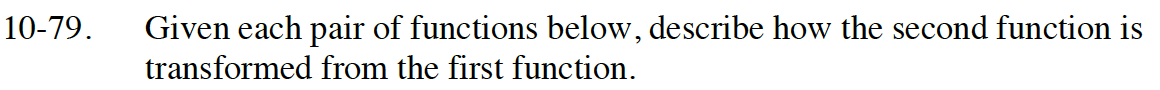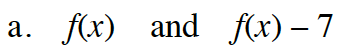Since f(x) is the y-coordinate, the transformation has each y-coordinate moved down 7 units.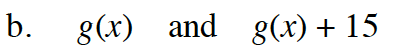Review hint (a):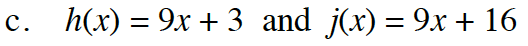What is the only difference between the two functions?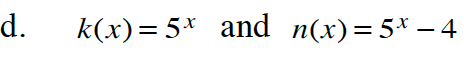n(x) is shifted down four units.